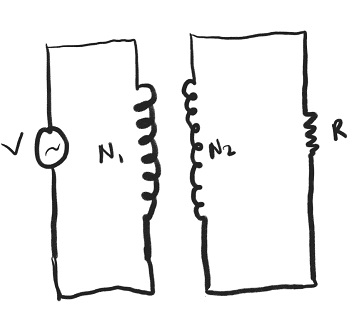# A transformer has N1 primary turns and N2 secondary turns. The primary coil is connected to an AC...

## Question:

A transformer has {eq}N_1 {/eq} primary turns and {eq}N_2 {/eq} secondary turns. The primary coil is connected to an AC source with voltage amplitude V. The secondary coil is connected to a resistor with resistance R. Let {eq}N_1 = 120, N_2 = 50~ V, V = 200 ~V, ~and~ R = 50~ \Omega {/eq}.Find the amplitude of the current {eq}I_2 {/eq} through the secondary coil.

A) 1.67 A

B) 16.0 A

C) 1.00 A

D) 0.50 A

## Need of Transformer:

All the electronic devices are made to operate on a certain range of voltage and current, and a higher or lower power supply can be harmful to those appliances. A transformer can change the incoming power supply to the required value of the power supply.

Become a Study.com member to unlock this answer! Create your account

Given Data

• The voltage in the primary coil is: {eq}V = 200\;{\rm{V}} {/eq}.
• The number of primary turns is: {eq}{N_1} = 120 {/eq}.
• The number of...